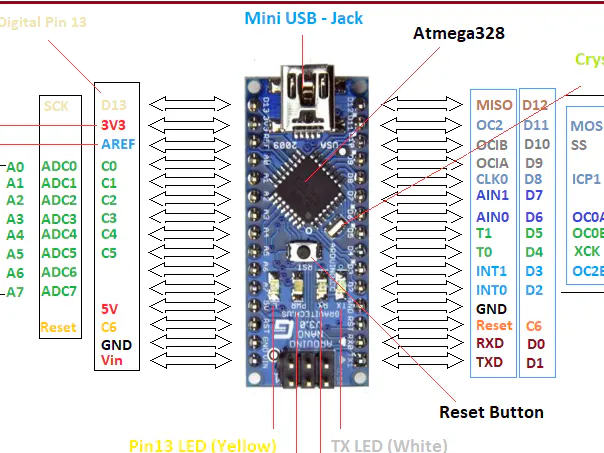# Random number generator © CC0

Generates a completely random number utilizing an unused pin and the in-built random number generator.

• 1,116 views
• 1 comment
• 1 respect

## Apps and online services

I made this by experimenting with the in-built random number generator and then once it was working, I then added the ability to take a floating pin and incorporate that into the equation, And as a result, we have the completely random number generator

## Code

##### random number generator codeC/C++
upload this to the arduino and open a serial monitor or plotter.
```long randomnum;  //creates the variable randomnum
int floatingpin = 0;  //creates the variable floatingpin
const int emptypin = A1;  //put an unused analog pin in here
void setup() {
Serial.begin(9600);  //initialises the serial port
randomSeed(23); //put any number in here
pinMode(emptypin, INPUT);  //sets emptypin as an input
}
void loop() {
floatingpin = analogRead(emptypin);  //sets floating pin to the value of the empty pin
randomnum = random(2,176);  //put a low number and a high number in here, they are the variables that set the parameters of the randomness.
Serial.println(randomnum);  //prints out the number
randomSeed(randomnum/(randomnum*(randomnum*floatingpin)));  //sets the seed to a comletely random number
delay(randomnum);  //waits for how long the random number is

}
```

## Schematics#### Published on

June 15, 2020
See similar projects
you might like

#### ANDI - Random Rhythm Generator - Electronics

Project tutorial by ANDinstruments

• 11,110 views
• 1 comment
• 30 respects

#### 8x8 LED Matrix Random Number Display

Project tutorial by scott mangiacotti

• 2,989 views
• 1 comment
• 5 respects

#### 10kHz to 120MHz VFO / RF Generator with Si5351 and Arduino

Project in progress by CesarSound

• 17,555 views
• 30 respects

#### DIY Simple Square Wave Generator Up to 1MHz

Project tutorial by Mirko Pavleski

• 11,044 views
• 24 respects

#### 10kHz to 225MHz VFO/RF Generator with Si5351 - Version 2

Project in progress by CesarSound

• 11,066 views